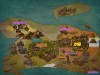# 星星灰暗着。# 星星灰暗着。

### 玄学算法/精彩DS总结 Vasuku

posted on 2018-09-18 17:58:20 | under Summary |

## $31.Complementary\ Graph$

1. 建立一个链表把所有点连起来，然后随便从链表中找一个点BFS。
2. 对于当前队首，将所有链表里没有与它连边且未被 $visit$ 的点 $push$ 进队列，并将它们从链表中删除并标记为 $visited$ 。
3. 重复此过程进行多次BFS直到链表为空。在删除一个点的时候就可以顺便统计信息了。

## $32.Segment\ Tree\ Dividing\&Conquering$

（时间）线段树分治是一种离线思想，是把处理目标的存在时间的区间映射到线段树上，再利用一个可撤销的数据结构进行整体地回答问题与处理影响。

$LOJ\ 121$ 动态图连通性

    void merge(int x,int y)
{
if(x==y)return;
if(dep[x]<dep[y])swap(x,y);
fa[y]=x;
s[++tp]=pi(y,dep[x]==dep[y]);
if(dep[x]==dep[y])++dep[x];
}
int find(int x){return fa[x]^x?find(fa[x]):x;}
void ruin(int x)
{while(tp>x)dep[fa[s[tp].fi]]-=s[tp].se,fa[s[tp].fi]=s[tp].fi,--tp;}

$BZOJ\ 4025$ 二分图

$[HAOI2017]$ 八纵八横

线段树分治傻题。
——zsyzsy

## $35.Hash$

ht[r]-ht[l-1]*mpow[r-l+1]

## $36.Fuka\ Ben/Fukua\ Beng$

$\huge\texttt{肥}_{\small\texttt{泵}^{\large\texttt{话}}}^{\large\texttt{克}_{\tiny\texttt{的}^{\small\texttt{说}}}}$ $\huge\texttt{一}_{\small\texttt{都}^{\large\texttt{不}}}^{\large\texttt{句}_{\small\texttt{信}\large\texttt{得}}}$

1.搜索树上线段树合并
$Beng$ 哥非常热爱线段树合并。
2.“这个题有好多枝可以剪！”
“这题有好多枝可以剪！”——oyiya
“剪什么枝？这题直接容斥就可以了。”——Beng

“ $Faker\_Beng$ 啊，你怎么不剪枝啊？”
” $\cdots\cdots$ 这个题有好多枝可以剪！“
3.“平衡树挺好的啊？”
“ $Beng$ 哥，我想把范围出高一点，但是出高一点要用 $Splay\cdots\cdots$ ”——Shenwei
“平衡树挺好的啊？” ——Beng
4.“（代码长度）小的就是大的，大的就是小的！”
5.“A是不可能让你A的。”
“ $Beng$ 哥，你看看 $result$ ，这题有多少人A掉？”——Shenwei
“ $A$ 是不可能让你 $A$ 的。”——Beng
6.“ $10^7\times 500$ 怎么会爆(int)啊？！”

“这题答案可能到多少啊？”——Shenwei
“哎呀不会爆不会爆， $10^7\times 500,5e8$ 怎么会爆啊？！”——Beng
7.“NOIp2018之前的一些想法”

长跑的目的不是更快，而是更强。 ------《强风吹拂》

8.僵硬 $Fake$
“弱肉强食 $\cdots\cdots$ ”——Shenwei
“我好弱的。”——Beng

“怎么说咧，无话可说。”——Shenwei
“肖大佬强得无话可说。”——Beng
9.当 $Faker$ 被问“你有没有 $A$ 一道题”且他已经 $A$ 时
“哎我都是抄（肖大佬）的。”/“大同小异大同小异。”——Beng
10.“你没改说明你不屑于改”
“肖大佬你肯定改了这道题”（ $fkb$ 指向一道 $zzq\ slide$ 里的神仙题）——Beng
“那要是我没改怎么办咧？”——Shenwei
“你没改说明你不屑于改。”——Beng
11.云玩家的嘲讽
“ $I\ Wanna$ 不随便玩。”
12 $Fake\ Beng$ 最爱的三样东西

“直接线段树合并”——Beng
“哎别用 $SA$ ，用 $SAM$ 就可以了”——Beng
“你就用 $\_find\_first,\_find\_next$ 优化一下这个过程”——Beng
13.成独分子
（盯着成都市第七中学的 $OIerDb$ ）原来成都和四川是一起算的名额啊！——Beng
14.传统艺能
$Faker\_Beng$ 热衷于 $OIerDb$ ，并每天都要用它来 $orz$ 机房的人和机房外的人。
15.对拍

## $37.Tournament$

$Landau's\ Theorem$

## $38.Derangement$

### 1. $O(n)$ 递推

$$f[n]=(n-1)(f[n-2]+f[n-1])$$

### 2. $O(n)$ 容斥

$$\sum_{i=0}^n(-1)^i\frac{n!}{i!}$$

### 3.继续扩展

$$dp[i]=(i-1)(dp[i-2]+dp[i-1])$$ 然后我们考虑 $j>0$ 的情况： $$dp[i][j]=dp[i-1][j-1]+dp[i][j-1]$$

### 4.变得更快

【话说感觉现在写总结越来越长越来越喜欢分阶段了啊